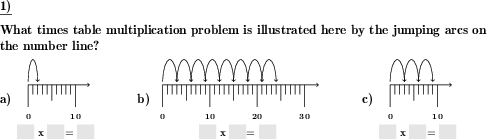Custom math worksheets at your fingertips# Details for problem "Number line illustrating times table problem"

Quickname: 6193

Elementary School, Primary School.

## Summary

On a numberline, 1 to 10 jumps each 1-10 units in length are depicted. What problem is shown and where do we land?

## Example## Description

A number line is shown. On the number line, a series of arcs illustrates a multiplication problem from a one to ten times table. For example, the product 5 x 9 is depicted by a series of five consecutive arcs starting at the zero position with each spanning nine units on the number line. The series ends at the number 45 on the number line, which is the result of this problem.

The task is to identify the multiplication problem shown and to read the result from the number line. The number of jumps and the width of each jump form the factors of the product required.

The number of problems is selectable. Problems will be randomly selected or a single times table, i.e. second factor, can be chosen.

To make the problem easier to solve, the second factor, which is equivalent to the width of the jumps, can be provided with the problem.

Download free printable worksheets for this math problem here. The worksheet contains the problems only, the solution sheet includes the answers. Just click on the respective link.

•Worksheet 1Solution sheet with answers
•Worksheet 2Solution sheet with answers
•Worksheet 3Solution sheet with answers

If you can not see the solution sheets for download, they may be filtered out by an ad blocker that you may have installed. If this is the case, please allow ads for this page and reload the page. The solution sheets will then reappear.

• Do these sample worksheets do not really fit?
• Do you need more math worksheets, with a different level of difficulty?
• Would you like to combine different problems on a worksheet and adjust them to your needs?
• As a teacher, you can put together your own worksheets using the automatically generated math problems provided.
With a free initial credit, you can start creating your own math worksheets in a few minutes.

You can try it for free! Register here, to create custom worksheets now!

## Customization options for this problem

Parameter
Possible values
Number of problems
1, 2, 3, 4, 5, 6
Number to practise
all, 1, 2, 3, 4, 5, 6, 7, 8, 9, 10
Provide times table factor
Yes, No